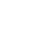Media Forums The Uganda Useless Elite Class Yoweri Museveni is the Biggest Parasite in Uganda Must Know : WHAT IS NATIONAL INCOME: BASIC CONCEPTS

#242

#### Must Know : WHAT IS NATIONAL INCOME: BASIC CONCEPTSNational Income is total amount of goods and services produced within the nation during the given period say, 1 year. It is the total of factor income i.e. wages, interest, rent, profit, received by factors of production i.e. labour, capital, land and entrepreneurship of a nation.
CONCEPTS OF NATIONAL INCOME:
There are various concepts of National Income, such as GDP, GNP, NNP, NI, PI, DI, and PCI which explain the facts of economic activities.
1. GDP at market price: Is money value of all goods and services produced within the domestic domain with the available resources during a year.
GDP = (P*Q)
Where,
GDP = gross domestic product
P = Price of goods and services
Q= Quantity of goods and services
GDP IS MADE UP OF 4 COMPONENTS:
(i) consumption
(ii) investment
(iii) government expenditure
(iv) net foreign exports of a country
GDP = C+I+G+(X-M)
Where,
C=Consumption
I=Investment
G=Government expenditure
(X-M) =Export minus import
2. GROSS NATIONAL PRODUCT (GNP): Is market value of final goods and services produced in a year by the residents of the country within the domestic territory as well as abroad. GNP is the value of goods and services that the country’s citizens produce regardless of their location.
GNP=GDP+NFIA or,
GNP=C+I+G+(X-M) +NFIA
Where,
C=Consumption
I=Investment
G=Government expenditure
(X-M) =Export minus import
NFIA= Net factor income from abroad.
3. NET NATIONAL PRODUCT (NNP) at MP: Is market value of net output of final goods and services produced by an economy during a year and net factor income from abroad.
NNP=GNP-Depreciation
or, NNP=C+I+G+(X-M) +NFIA- IT-Depreciation
Where,
C=Consumption
I=Investment
G=Government expenditure
(X-M) =Export minus import
NFIA= Net factor income from abroad.
IT= Indirect Taxes
4. NATIONAL INCOME (NI): Is also known as National Income at factor cost which means total income earned by resources for their contribution of land, labour, capital and organisational ability. Hence, the sum of the income received by factors of production in the form of rent, wages, interest and profit is called National Income.
Symbolically,
NI=NNP +Subsidies-Interest Taxes
or, GNP-Depreciation +Subsidies-Indirect Taxes
or, NI=C+G+I+(X-M) +NFIA-Depreciation-Indirect Taxes +Subsidies
5. PERSONAL INCOME (PI): Is the total money income received by individuals and households of a country from all possible sources before direct taxes. Therefore, personal income can be expressed as follows:
PI=NI-Corporate Income Taxes-Undistributed Corporate Profits- Social Security Contribution +Transfer Payments.
6. DISPOSABLE INCOME (DI) : It is the income left with the individuals after the payment of direct taxes from personal income. It is the actual income left for disposal or that can be spent for consumption by individuals.
Thus, it can be expressed as:
DI=PI-Direct Taxes
7. PER CAPITA INCOME (PCI): Is calculated by dividing the national income of the country by the total population of a country.
Thus, PCI=Total National Income/Total National Population
8. MEASUREMENT OF NATIONAL INCOME:
There are three methods to calculate National Income:
(i) Income Method
(iii) Expenditure Method
(i) INCOME METHOD
In this National Income is measured as flow of income.
We can calculate NI as:
NET NATIONAL INCOME = Compensation of Employees+ Operating surplus mixed (w +R +P +I) + Net income + Net factor income from abroad.
Where,
W = Wages and salaries
R = Rental Income
P = Profit
I = Mixed Income